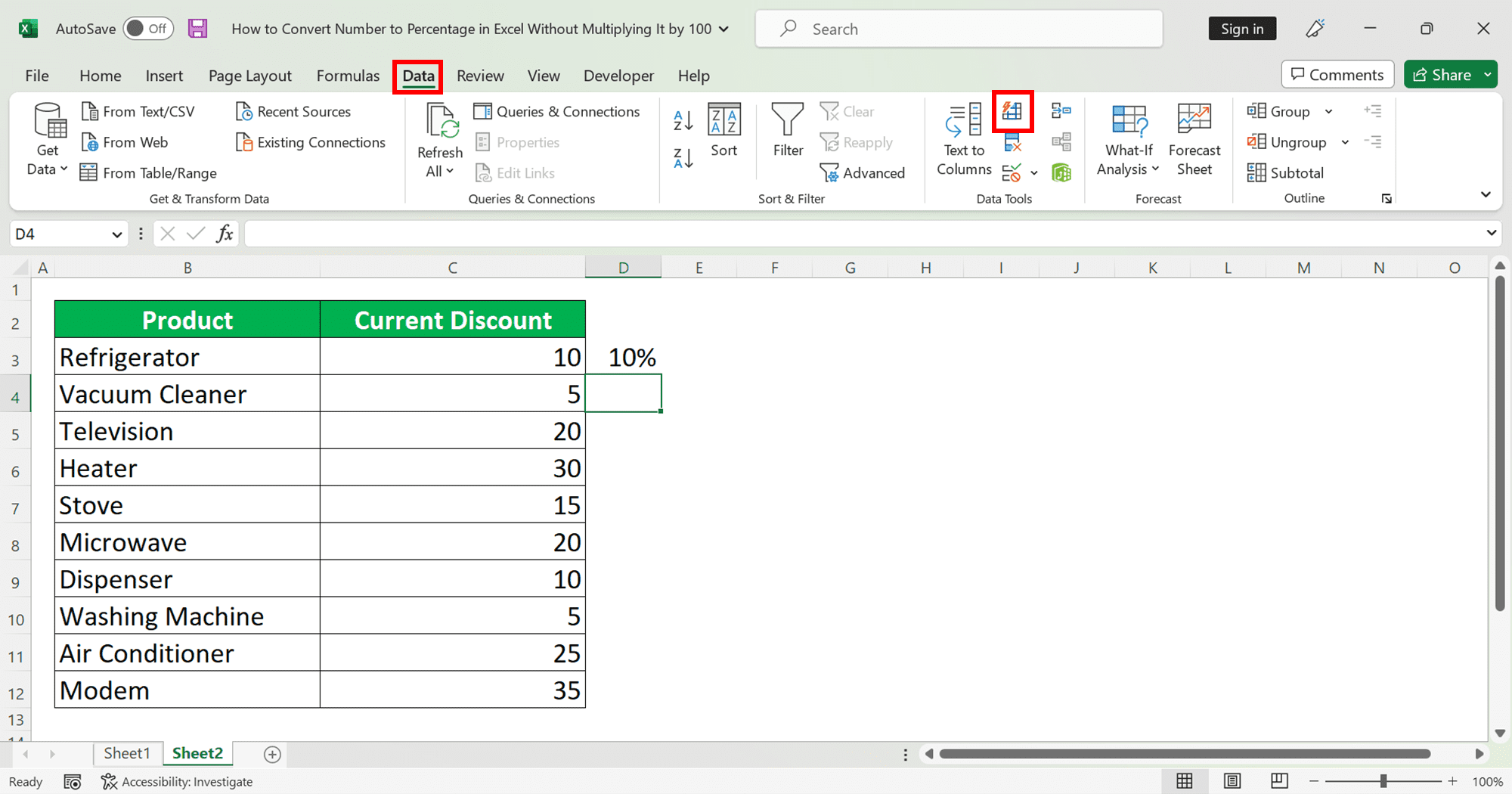How to Convert Number to Percentage in Excel Without Multiplying It by 100 - Compute Expert

# How to Convert Number to Percentage in Excel Without Multiplying It by 100

Home >> Excel Tutorials from Compute Expert >> Excel Tips and Trick >> How to Convert Number to Percentage in Excel Without Multiplying It by 100

From this tutorial, you will learn how to convert numbers to percentages in Excel without multiplying them by 100.

In Excel, we sometimes have numbers in our data tables that we want to convert to percentages. However, we might not want to multiply those numbers by 100 to be able to do the conversion like what the conversion process in Excel usually does to our numbers. This is often the problem we face when we get percentage numbers from external sources and they are formatted as pure numbers although they are percentages.

To just append a percentage symbol to our numbers without having to go through the multiplication, we need to understand the correct methods to be able to do that. In this tutorial, we will discuss those methods that we can utilize to do the conversion to percentages while neglecting the usual automatic multiplication by 100.

Want to know what are the methods and how we can implement them? Let’s get into it right away.

Disclaimer: This post may contain affiliate links from which we earn commission from qualifying purchases/actions at no additional cost for you. Learn more

## How to Convert Number to Percentage in Excel Without Multiplying It by 100 1: Custom Format

Now, let’s discuss the first method to append a percentage symbol to our numbers without multiplying it by 100 in Excel. This first method uses the “Format Cells” feature that is available in Excel, particularly its custom format.

Keep in mind though that this method will only change the display of your number into a percentage format while still keeping its value intact. That means if, for example, you convert 100 to 100% using this method and you multiply the 100% with 100, the result will be 10000 (100 multiplied by 100) not 100 (100 multiplied by 100%).

Here are the detailed steps to implement this method.

1. Highlight all the cells with the numbers that you want to convert to percentage format2. Go to the “Home” tab on your ribbon and click on the “Number” button. Alternatively, you can just press Ctrl + 1 on your keyboard (Command + 1 on Mac)3. Select the “Custom” category by clicking on it4. In the “Type” text box on the right, type 0\%. Add decimal places behind the 0 if necessary (to force two decimal places on your numbers, type 0.00\%. To add optional three decimal places on your numbers, type 0.###\%)5. Click the “OK” button6. Done! The % symbol has been appended on your numbers## How to Convert Number to Percentage in Excel Without Multiplying It by 100 2: Flash Fill

The first method only changes the appearance of our numbers into their percentage format without changing their actual values. Now, what if we have many cells in a column with number values and we want to convert them all to percentages with their actual values too? You may want to use the “Flash Fill” feature in Excel for that.

“Flash Fill” is a great feature in Excel that can mimic our data input pattern and apply it to make our data input done much faster. We can also use it to make us convert our numbers into percentages much more automatically.

Here are the detailed steps to use the feature to do our conversion process.

1. On the cell on the right of the first number that we want to convert into a percentage in a column, type its percentage form (the number followed by a “%” symbol) and press Enter2. Go to the “Data” tab on your ribbon and click the “Flash Fill” button there or just press Ctrl and E buttons simultaneously on your keyboard3. All your numbers have been converted into percentages on the column on the right of the column of your numbers! To make the percentages replace the numbers in your column, just copy them there and delete the percentages on the right4. Done! All your numbers have been converted into their percentage format!To know more about this “Flash Fill” feature in Excel, what it can do and how to utilize it optimally, you can read this tutorial.

## Exercise

After you have learned how to convert numbers to percentages in Excel without having to multiply them by 100, let’s do a simple exercise to sharpen your understanding.

Open an Excel file and fill a column up with pure numbers. Then, try to convert them into percentages without multiplying them by 100 by using the two methods above. Try each method so you get a better grasp of them.

How is it? Can you do the conversion? If you can’t, go back to the tutorial steps again to see what you should have done better on the implementation of the methods.

If the number you want to convert to a percentage is from a formula and you want to preserve the formula and use the percentage for calculation, then you still need to use the standard method to do the conversion from a number to a percentage in Excel (change the data format to percentage through the “Format Cells” dialog box, click “Percent Style” button, etc).

To keep the value of the number to before it is multiplied by 100, you can just divide the formula result by 100 before you convert the number. Doing it by adding the division process to the formula might be preferable to simplify your data processing.

Excel tutorials you might want to read too:

Get updated excel info from Compute Expert by registering your email. It's free!